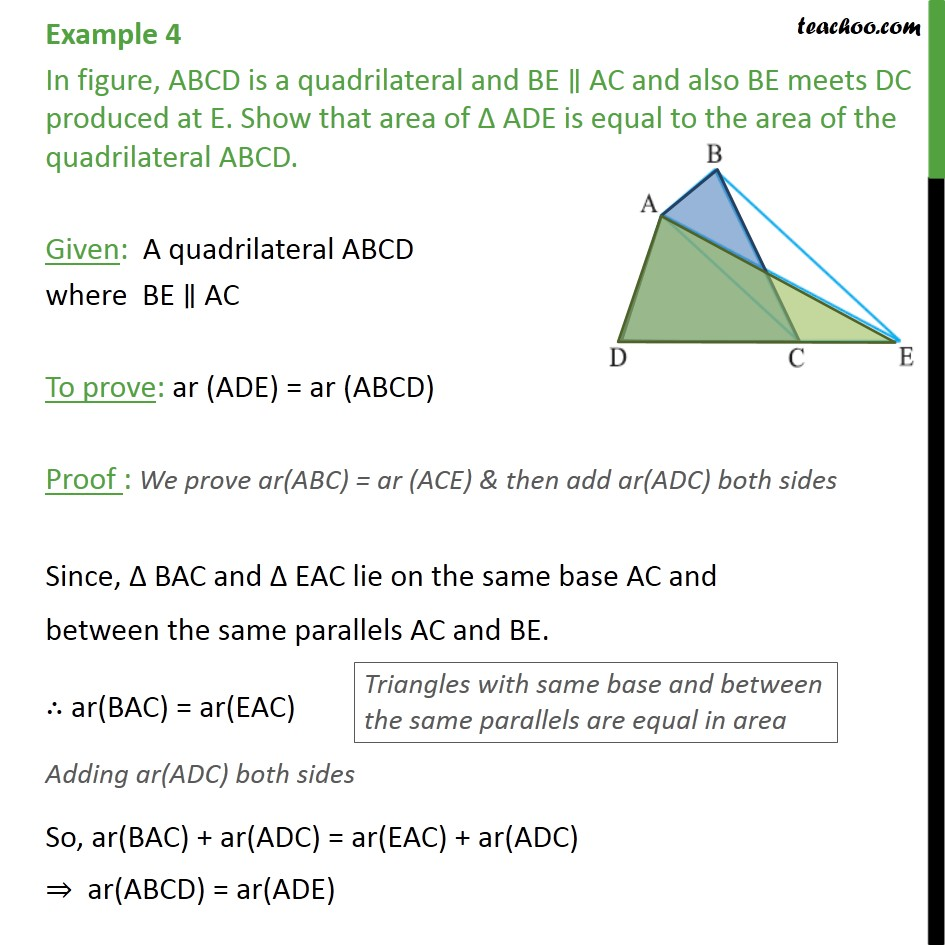1. Chapter 9 Class 9 - Areas of Parallelograms and Triangles (Deleted)
2. Serial order wise
3. Examples

Transcript

Example 4 In figure, ABCD is a quadrilateral and BE ∥ AC and also BE meets DC produced at E. Show that area of Δ ADE is equal to the area of the quadrilateral ABCD. Given: A quadrilateral ABCD where BE ∥ AC To prove: ar (ADE) = ar (ABCD) Proof : We prove ar(ABC) = ar (ACE) & then add ar(ADC) both sides Since, Δ BAC and Δ EAC lie on the same base AC and between the same parallels AC and BE. ∴ ar(BAC) = ar(EAC) Adding ar(ADC) both sides So, ar(BAC) + ar(ADC) = ar(EAC) + ar(ADC) ⇒ ar(ABCD) = ar(ADE)